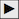# Dilation Challenges: The Dilation Family

In this activity you will compare the motion of a point to the motion of its dilated image. (List items in bold below contain questions for you to answer, as you work to analyze and understand your results.)

### Dilate a Point

Begin by showing a point and its dilated image, and describing how the dilation behaves.

1. Show independent variable x and drag it around. This is the independent variable.
2. Show the center point C and the scale parameter s.
3. Dilate x and drag x again. The blue point depends on x, so we call it the dependent variable.
4. Show the dependent variable’s label.
You can read DC,s(x) as “the dilation of x about C by scale factor s.”
5. Drag x up. How does DC,s(x) move? Drag x left. How does DC,s(x) move? In what direction, and how fast?
6. Turn on tracing for both variables. Drag independent point x to trace out an interesting shape.
7. Describe the traced shapes. How are they similar, and how are they different? Consider position, size, angle, and anything else you think of. In your answer include a drawing or screen capture of your two traced shapes.
8. Erase the traces and trace a new shape that goes through the center point.
9. What happened when you went through the center of dilation? Describe these traced shapes, and include a drawing or screen capture in your answer. Were there any fixed points? If so, where?
A location where x and DC,s(x) come together is called a fixed point of the function.

### Use a Different Scale Factor

1. Click parameter s and change its value to 2.00.
If the dependent variable disappears, you may have to drag x or C to make it reappear.
2. Drag x straight up. Which way does DC,s(x) go, and how fast? Drag x left. Which way does DC,s(x) go, and how fast?
3. Drag point x to make a shape.
4. How are these shapes different from the shapes you made when you dilated by 0.50? In your answer describe the traced shapes and include a drawing or screen capture.
5. Can you drag the two points together to find a fixed point? If so, where is it?
6. Change s to 1.00, erase the traces, and then drag x. What happens? Where are the fixed points?
7. Change s to –1.00, erase the traces, and then drag x. What happens when the scale factor is negative?
8. Predict what will happen (a) if s is –2.00, (b) if s is very small, and (c) if s = 0. Explain and draw your predictions first, before you test them.

### Restrict the Domain

Now you’ll restrict point x to a polygon and observe the effect on DC,s(x).
1. Click thepointer to go to page 2 of the sketch.
2. Drag x and observe the behavior of DC,s(x).
3. Restrict x to the polygon by pressing Restrict x to polygon. Drag x again.
4. Describe the function’s domain. In other words, where can you drag the independent variable? A domain like this is called a restricted domain.
5. Press Animate x to move x around its domain. As x varies, DC,s(x) traces out the range.
6. How does the range compare to the restricted domain? What features of the domain and range are similar, and what features are different?

### Dilation Challenges

Now you’ll have a chance to try some dilation challenges. Some will be harder than others; if you accomplish them all, you'll be a dilation function expert!

1. Click the pointerto go to the first challenge.
2. For each challenge page, describe clearly how you solved it, and include a drawing or screen capture. Describe any shortcuts you invented to make the challenge easier.